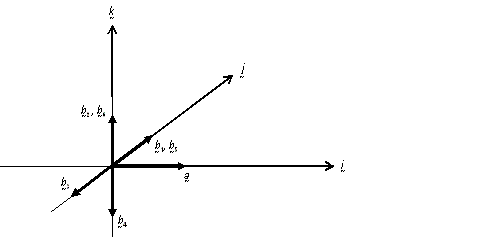#### You may also likeA quadrilateral changes shape with the edge lengths constant. Show the scalar product of the diagonals is constant. If the diagonals are perpendicular in one position are they always perpendicular?### A Knight's Journey

This article looks at knight's moves on a chess board and introduces you to the idea of vectors and vector addition.### 8 Methods for Three by One

This problem in geometry has been solved in no less than EIGHT ways by a pair of students. How would you solve it? How many of their solutions can you follow? How are they the same or different? Which do you like best?

# V-P Cycles

##### Age 16 to 18 Challenge Level:

Derek Wan gave an excellent solution and we include his diagram of the final result at the end. Here's a solution from Thomas Lauffenberger who finally reveals that he is an American.

Suppose the vector product ${\bf a} \times {\bf b}\neq {\bf 0}$. Define a sequence of vectors ${\bf b_0},\ {\bf b_1},\ {\bf b_2}\ldots$ by ${\bf b_0}={\bf b}$ and ${\bf b_{n+1}}={\bf a}\times {\bf b_n}$

#### Part 1:

Show that ${\bf b_n} \rightarrow 0$ as $n \rightarrow \infty$ if ${\bf |a|} < 1$. \par According to the article supplied on the basics of vector multiplication, the resulting vector is perpendicular to its parents and has a magnitude of ${\bf |v_1||v_2|} \sin \theta$. In the problem posed, we are given that ${\bf |a|}$ is less than 1. The maximum value of $\sin \theta$ is 1, so the product ${\bf |a|}\sin \theta$ must be less than 1; the magnitudes of the succeeding vectors in the sequence given by ${\bf b_{n+1}}={\bf a}\times {\bf b_n}$ decrease as a geometric series, so they will tend to 0 as $n$ tends to infinity. The only vector with a magnitude off 0 is the zero vector, which ${\bf b_n}$ will tend to as $n$ tends to infinity.

#### Part 2:

Here $|{\bf a}|=1$ and $|{\bf b_1}|=r$. The supplied hint suggests using ${\bf a} = {\bf i}$ and ${\bf b_1}= r{\bf j}$. Doing the cross product matrix math (a $3 \times 3$ matrix with "${\bf i}, {\bf j}, {\bf k}$" on the top line, "$1,0,0$" for the ${\bf a}$ line, and "$0,r,0$" for ${\bf b_1}$, we obtain ${\bf b_2}= r{\bf k}$. Performing the next cross product, ${\bf a} \times {\bf b_2}$, we obtain $-r{\bf j}$, and, doing it again, $-r{\bf k}$. The cross product ${\bf a}\times {\bf b_4}$ produces a result of $r{\bf j}$ which is ${\bf b_1}$; therefore, we have a cycle.

If we begin our movement from the origin in 3-space, ${\bf b_1}$ tells us to advance $r$ units up the $y$-axis ${\bf j}$ direction). Then ${\bf b_2}$ says to advance $r$ units positively along the $z$-axis. Vectors ${\bf b_3}$ and ${\bf b_4}$, respectively, move again along the $y$ and $z$ axes, but now $r$ units in the negative direction. The shape that follows is a square with sides of $r$ units, located within the $yz$-plane in this instance. I'm sure that much more can be said about this, regarding our choices for ${\bf a}$ and ${\bf b}$; I'll do more work on it and post back more ideas. \par Addendum to previous submission- The key components determining the location of the square are ${\bf a}$, a unit vector, and $\bf {b}$, a vector of magnitude $r$. Vector ${\bf a}$ determines the plane in which the square will situate itself which has vector ${\bf a}$ perpendicular to the plane of the square; ${\bf b}$ moves within that plane. With the direction vector ${\bf a}$ pointed up relative to our perspective, the motion of the vector ${\bf b}$ is to trace the square in a counterclockwise direction, like a baseball diamond. (Yes, I'm an American!)

Thomas is adding one vector onto the previous one to generate a square. Here is Derek Wan's sketch of all 6 vectors:We can see that the vectors lie on the $y$-$z$ plane perpendicular to the vector ${\bf a}$.